SPSS TUTORIALS BASICS ANOVA REGRESSION FACTOR CORRELATION

# SPSS Paired Samples T-Test Tutorial

A paired samples t-test examines if 2 variables
are likely to have equal population means.

## Example

A teacher developed 3 exams for the same course. He needs to know if they're equally difficult so he asks his students to complete all 3 exams in random order. Only 19 students volunteer. Their data -partly shown below- are in compare-exams.sav. They hold the number of correct answers for each student on all 3 exams.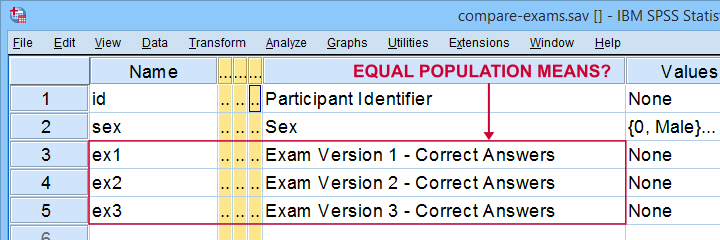## Null Hypothesis

Generally, the null hypothesis for a paired samples t-test is that 2 variables have equal population means. Now, we don't have data on the entire student population. We only have a sample of N = 19 students and sample outcomes tend to differ from population outcomes. So even if the population means are really equal, our sample means may differ a bit. However, very different sample means are unlikely and thus suggest that the population means aren't equal after all. So are the sample means different enough to draw this conclusion? We'll answer just that by running a paired samples t-test on each pair of exams. However, this test requires some assumptions so let's look into those first.

## Paired Samples T-Test Assumptions

Technically, a paired samples t-test is equivalent to a one sample t-test on difference scores. It therefore requires the same 2 assumptions. These are

1. independent observations;
2. normality: the difference scores must be normally distributed in the population. Normality is only needed for small sample sizes, say N < 25 or so.

Our exam data probably hold independent observations: each case holds a separate student who didn't interact with the other students while completing the exams.
Since we've only N = 19 students, we do require the normality assumption. The only way to look into this is actually computing the difference scores between each pair of examns as new variables in our data. We'll do so later on.

At this point, you should carefully inspect your data. At the very least, run some histograms over the outcome variables and see if these look plausible. If necessary, set and count missing values for each variable as well. If all is good, proceed with the actual tests as shown below.

## SPSS Paired Samples T-Test Dialogs

You find the paired samples t-test under AnalyzeCompare MeansPaired Samples T Test as shown below.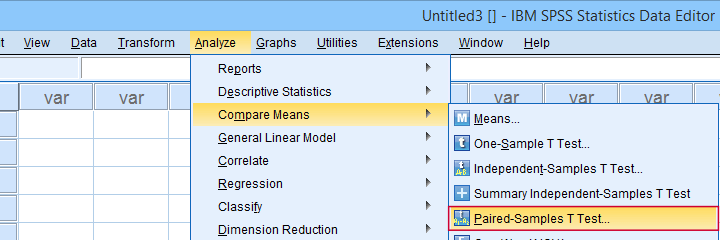In the dialog below,select each pair of variables andmove it to “Paired Variables”. For 3 pairs of variables, you need to do this 3 times.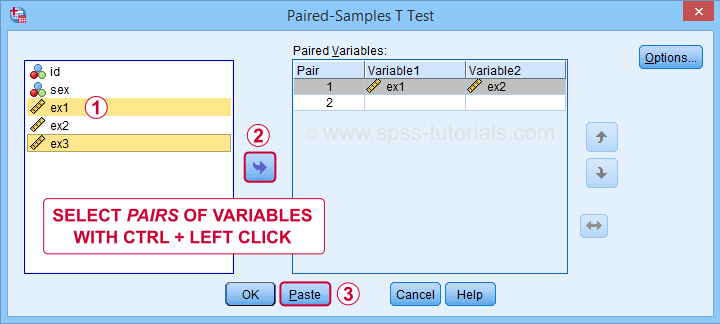Clicking creates the syntax below. We added a shorter alternative to the pasted syntax for which you can bypass the entire dialog. Let's run either version.

## Paired Samples T-Test Syntax

*Syntax pasted from analyze - compare means - paired-samples t-test.

T-TEST PAIRS=ex1 ex1 ex2 WITH ex2 ex3 ex3 (PAIRED)
/CRITERIA=CI(.9500)
/MISSING=ANALYSIS.

*Shorter version below results in exact same output.

T-TEST PAIRS=ex1 to ex3
/CRITERIA=CI(.9500)
/MISSING=ANALYSIS.

## Paired Samples T-Test Output

SPSS creates 3 output tables when running the test. The last one -Paired Samples Test- shows the actual test results.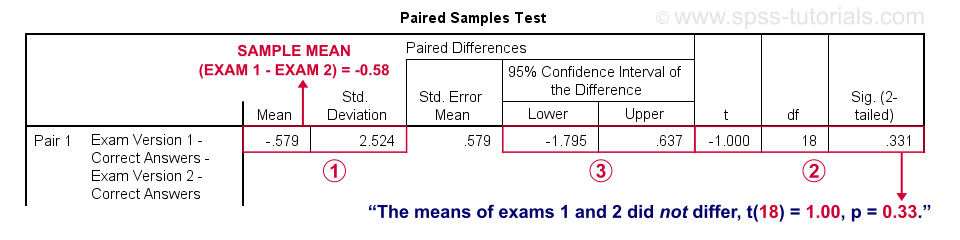SPSS reports the mean and standard deviation of the difference scores for each pair of variables. The mean is the difference between the sample means. It should be close to zero if the populations means are equal.The mean difference between exams 1 and 2 is not statistically significant at α = 0.05. This is because ‘Sig. (2-tailed)’ or p > 0.05.The 95% confidence interval includes zero: a zero mean difference is well within the range of likely population outcomes.

In a similar vein, the second test (not shown) indicates that the means for exams 1 and 3 do differ statistically significantly, t(18) = 2.46, p = 0.025. The same goes for the final test between exams 2 and 3.

## Effect Size - Cohen’s D

Our t-tests show that exam 3 has a lower mean score than the other 2 exams. The next question is: are the differences large or small? One way to answer this is computing an effect size measure. For t-tests, Cohen’s D is often used. Sadly, SPSS 27 is the only version that includes it. However, it's easily computed in Excel as shown below.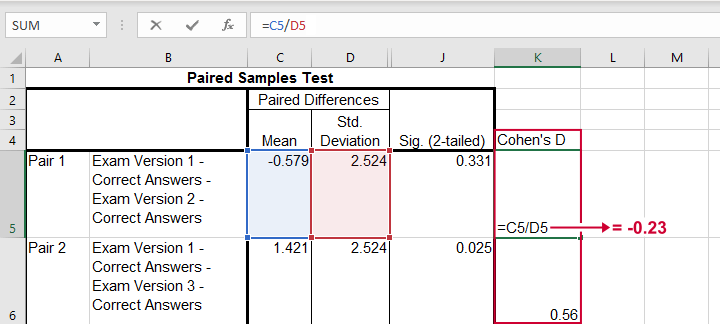The effect sizes thus obtained are

• d = -0.23 (pair 1) - roughly a small effect;
• d = 0.56 (pair 2) - slightly over a medium effect;
• d = 0.57 (pair 3) - slightly over a medium effect.

## Interpretational Issues

Thus far, we compared 3 pairs of exams using 3 t-tests. A shortcoming here is that all 3 tests use the same tiny student sample. This increases the risk that at least 1 test is statistically significant just by chance. There's 2 basic solutions for this:

If you choose the ANOVA approach, you may want to follow it up with post hoc tests. And these are -guess what?- Bonferroni corrected t-tests again...

## Testing the Normality Assumption

Thus far, we blindly assumed that the normality assumption for our paired samples t-tests holds. Since we've a small sample of N = 19 students, we do need this assumption. The only way to evaluate it, is computing the actual difference scores as new variables in our data. We'll do so with the syntax below.

*Compute difference scores as new variables.

compute dif_1_2 = ex1 - ex2.
compute dif_1_3 = ex1 - ex3.
compute dif_2_3 = ex2 - ex3.
execute.

## Result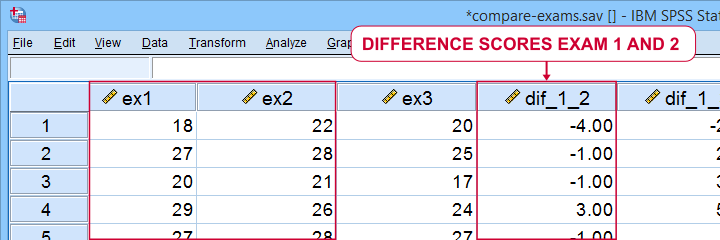We can now test the normality assumption by running

on our newly created difference scores. Since we discussed both tests in separate tutorials, we'll limit ourselves to the syntax below.

*Run normality tests from Analyze - Descriptive Statistics - Explore.

EXAMINE VARIABLES=dif_1_2 dif_1_3 dif_2_3
/statistics none
/plot npplot.

*Note: difference score between 1 and 2 violates normality assumption.

## Result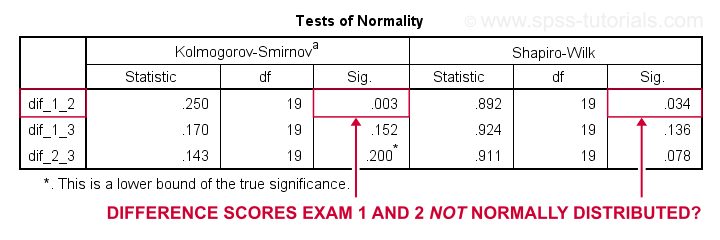Conclusion: the difference scores between exams 1 and 2 are unlikely to be normally distributed in the population. This violates the normality assumption required by our t-test. This implies that we should perhaps not run a t-test at all on exams 1 and 2. A good alternative for comparing these variables is a Wilcoxon signed-ranks test as this doesn't require any normality assumption.

Last, if you compute difference scores, you can circumvent the paired samples t-tests altogether: instead, you can run one-sample t-tests on the difference scores with zeroes as test values. The syntax below does just that. If you run it, you'll get the exact same results as from the previous paired samples tests.

*Test if all difference scores have zero means.

T-TEST
/TESTVAL=0
/MISSING=ANALYSIS
/VARIABLES=dif_1_2 dif_1_3 dif_2_3
/CRITERIA=CI(.95).

Right, so that'll do for today. Hope you found this tutorial helpful. And as always:

# Tell us what you think!

*Required field. Your comment will show up after approval from a moderator.

# THIS TUTORIAL HAS 19 COMMENTS:

•### By dr muhamad ali rahni on September 2nd, 2018

I'm not into statistics but I do supervise MS students. Your interpretation of SPSS output on repeated measures is by far the best. Thanx from K.L. Msia!

•### By Teshale Tefera on February 19th, 2019

It is good way of instructing and it is better if you test your customers knowledge and skills on different areas of higher statistics supplemented with certificate. I expect.

•### By Naod on January 9th, 2020

well explained!

•### By Naod on January 9th, 2020

very good explanation

•excellent